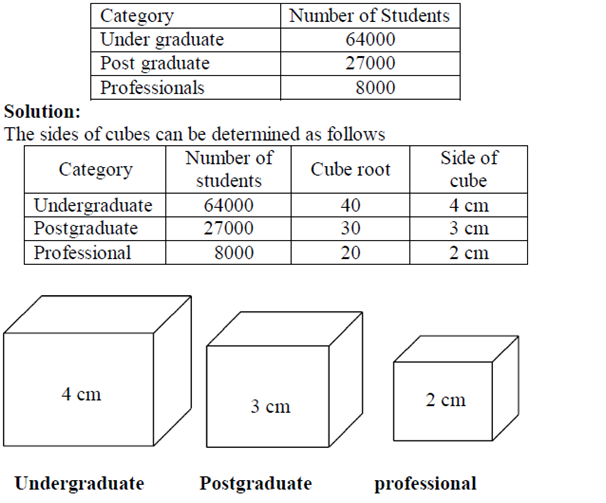# Statistics for SSC-CGL Tier-II (Paper-III) – Part-10

By | November 20, 2016

DIAGRAMATIC AND GRAPHICAL REPRESENTATION:

TYPES OF DIAGRAMS:

Three-dimensional Diagrams:

Three-dimensional diagrams, also known as volume diagram, consist of cubes, cylinders, spheres, etc. In such diagrams three things, namely length, width and height have to be taken into account. Of all the figures, making of cubes is easy. Side of a cube is drawn in proportion to the cube root of the magnitude of data.

Cubes of figures can be ascertained with the help of logarithms. The logarithm of the figures can be divided by 3 and the antilog of that value will be the cube-root.

Example 9:

Represent the following data by volume diagram.Pictograms and Cartograms:

Pictograms are not abstract presentation such as lines or bars but really depict the kind of data we are dealing with. Pictures are attractive and easy to comprehend and as such this method is particularly useful in presenting statistics to the layman. When Pictograms are used, data are represented through a pictorial symbol that is carefully selected.

Cartograms or statistical maps are used to give quantitative information as a geographical basis. They are used to represent spatial distributions. The quantities on the map can be shown in many ways such as through shades or colours or dots or placing pictogram in each geographical unit.